﻿ Direct numerical simulation of turbulence at periodic boundaries - XSim

# Direct numerical simulation of turbulence at periodic boundaries

Update: June 1, 2017
OpenFOAM 4.x

$FOAM_TUTORIALS/DNS/dnsFoam/boxTurb16 ## Summary The flow in a cube that all six sides are periodic boundaries will be solved until 10 seconds without using a turbulence model. The turbulence generated by the utility boxTurb is used as the initial condition, and after the calculation, the enstrophyis calculated with the utility postProcess. The mesh and initial conditions are as follows. The analysis area is divided into 16 equal parts in each of the X, Y, and Z directions as mesh.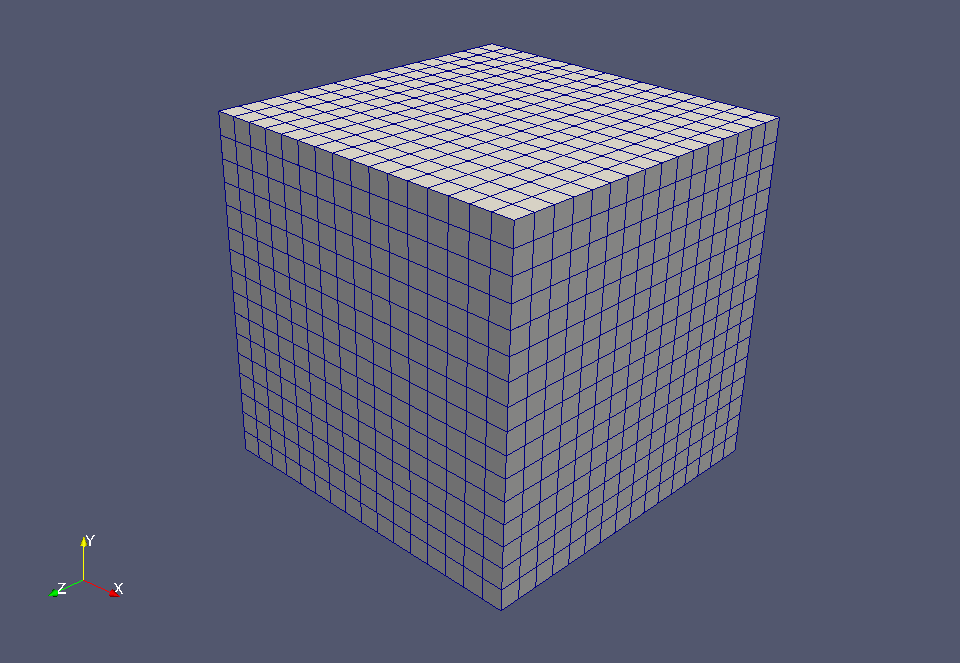Meshes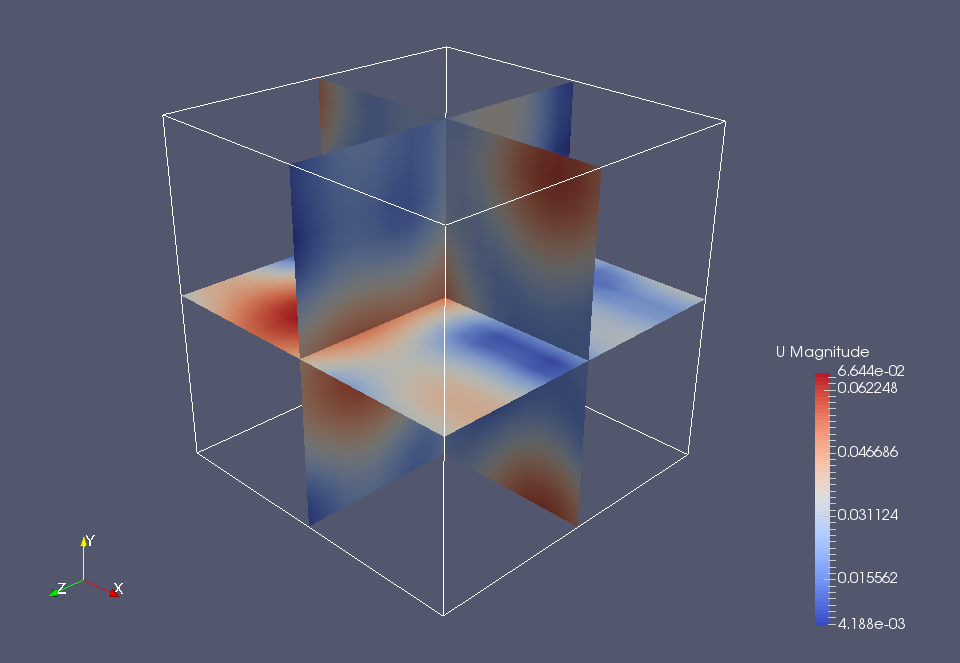Initial velocity (U) After the potential flow calculation, the enstrophy is calculated and output. To visualize the enstrophy, check the "enstrophy" in the "Properties" tab in ParaView.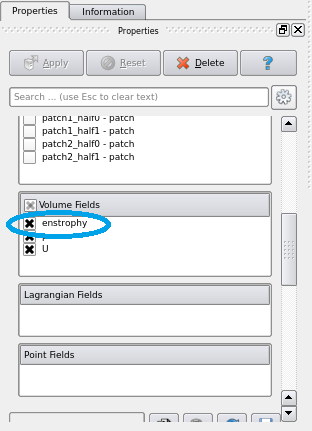Check "enstrophy" The calculation result is as follows. Absolute value of flow velocity (U)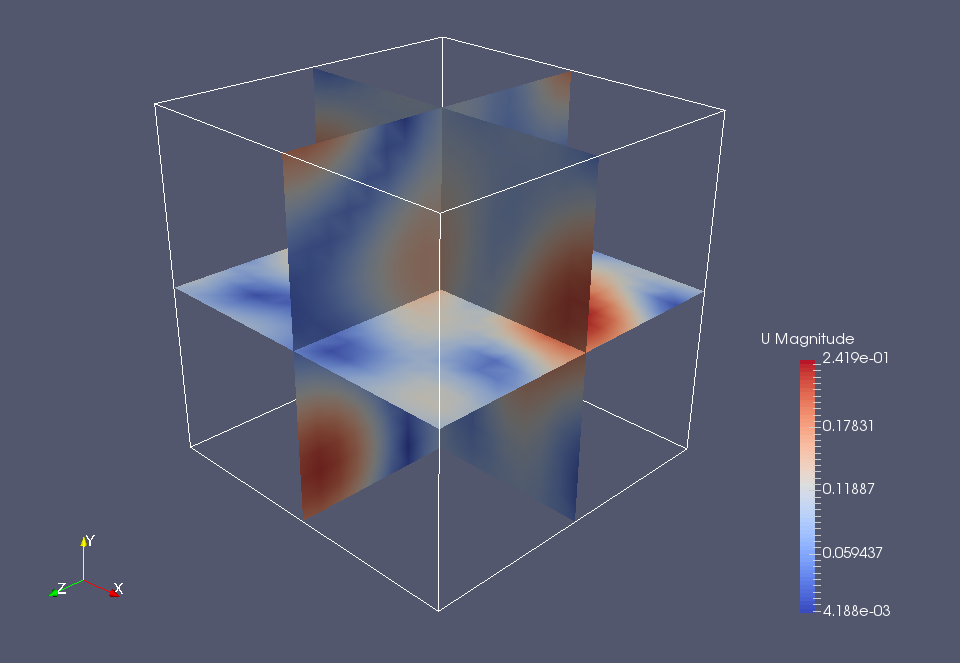Absolute value of flow velocity at 10 seconds (U)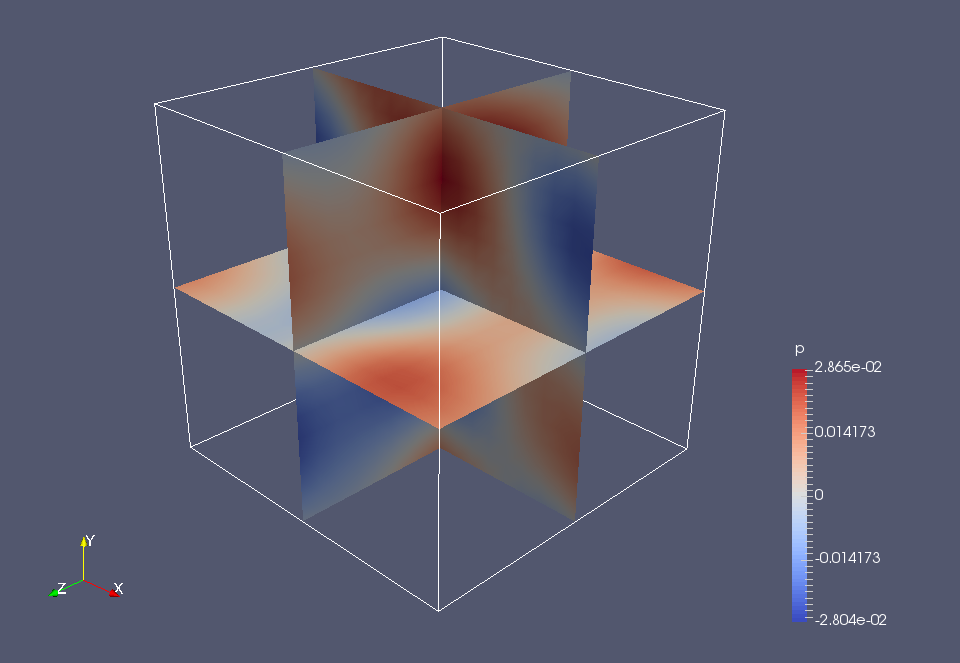Pressure at 10 seconds (p)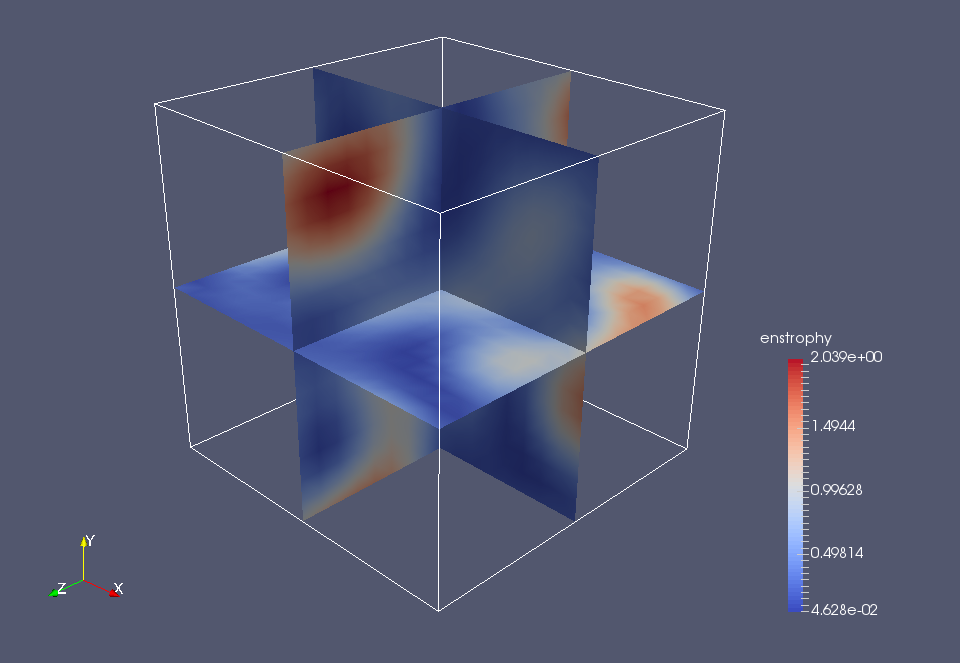Enstrophy at 10 seconds Since all boundary surfaces are periodic boundaries, momentum is conserved throughout the system and the flow does not decay. ## Commands cp -r$FOAM_TUTORIALS/DNS/dnsFoam/boxTurb16 boxTurb16
cd boxTurb16

blockMesh
boxTurb
dnsFoam
postProcess -func enstrophy

paraFoam

The boxTurb generates the initial conditions, and the postProcess calculates the enstrophy.

## Calculation time

--- *Single, Core(TM) i7-2600 CPU @ 3.40GHz 3.40GHz